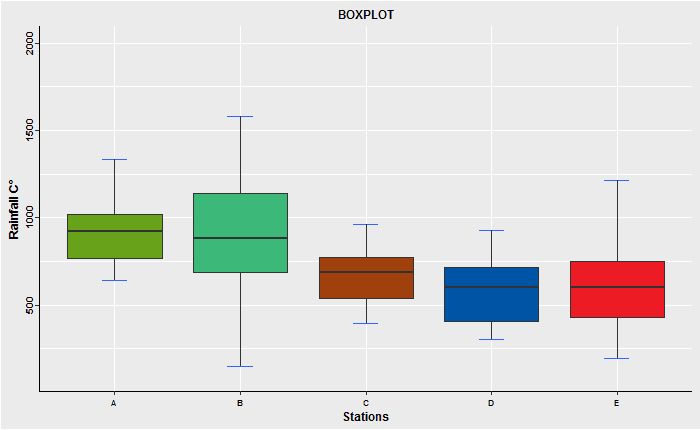# Creating boxplot in rstudio

## Creating boxplot in rstudioThe term "box plot" comes from the fact that the graph looks like a rectangle with lines extending from the top and bottom. Because of the extending lines, this type of graph is sometimes called a box-and-whisker plot. In descriptive statistics, a boxplot is a method for graphically depicting groups of numerical data through their quartiles.

A box plot is a graphical rendition of statistical data based on the minimum (Q0 or 0 th percentile), first quartile (Q1 or 25 th percentile), median (Q2 or 50 th percentile), third quartile (Q3 or 75 th percentile), and maximum (Q4 or 100 th percentile).

>>> How to make Box plot in R Studio?, How do you make a Boxplot in R studio?, How do I create a box plot?, Creating Boxplots in RStudio, ggplot2 box plot, R boxplot, Creating Boxplots in RStudio, Boxplot with ggplot2.

In this course I’ll show you how to create an amazing boxplot using RStudio.

### RScript

Raifall <- data.frame(BOX_PLOT_DATA)
PLOT.DATA <- gather(Raifall, "x", "y")

BOX1 <- ggplot(PLOT.DATA, aes(x, y)) +
labs(x = "Stations", y = "Rainfall mm") +
ylim(100, 2000)+
stat_boxplot(geom = 'errorbar', width = 0.2, colour= "red") +
ggtitle("BOXPLOT")+
geom_boxplot(fill = "gray", alpha = 1) +
theme(axis.title.x = element_text(color = "#000000", size = 9,
face = "bold", hjust = 0.5, vjust = 1),
axis.title.y = element_text(color = "#000000", size = 9,
face = "bold", hjust = 0.5, vjust = 1),
plot.title = element_text(color = "#000000", size = 12,
face = "bold", hjust = 0.5, vjust = 1)) +
theme(axis.text.x = element_text(color = "#000000", size = 9, angle = 0,
face = "bold", hjust = 0.5, vjust = 1),
axis.text.y = element_text(color = "#000000", size = 7, angle = 90,
face = "bold", hjust = 0.5, vjust = 1),
axis.line = element_line(color = "green", size = 1,
linetype= "solid")
)
BOX1

### Theme boxplot

### theme_dark() ### theme_bw() ### theme_classic()
### theme_gray() default theme
### theme(plot.background = element_rect(fill = '#ebebeb'))
### geom_boxplot(fill = '#0000ff', alpha = 0.5)
### geom_boxplot(fill = c('#cbb99d', '#0000ff', '#00ff00', '#ffff00','#0000ff'), alpha = 1)
### geom_boxplot(fill = c('#68a11a', '#3cb878', '#a0410d', '#0054a6','#ed1c24'), alpha = 1)